# QlikView App Dev

Discussion Board for collaboration related to QlikView App Development.

Announcements
cancel
Showing results for
Did you mean: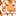Creator

## Expression help

I have attached the sample excel and the QVW I am trying to work out...

I need some help with the expression calculation...any help is appreciated...I have my request in QVW.

Thanks much.

10 Replies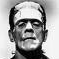Master II

See the attached qvw. !Creator II

Hi

Try below Expression

sum(TOTAL [Adj Net Size - SF])-sum(TOTAL {<[Occupying Depts]={'Unallocated'}>}[Adj Net Size - SF])*sum([SF Percentage])Champion III

Check this?

Sorry use this expr:

= Num((sum(TOTAL [Adj Net Size - SF]) - sum(TOTAL {< [Occupying Depts] = {'Unallocated'} >} [Adj Net Size - SF])) , '###0') * Subfield([SF Percentage], '%',1)Creator II

Hi,

Try Below expression with Paranthesis

(sum(TOTAL {<[Occupying Depts]={'Unallocated'}>}[Adj Net Size - SF]))*sum([SF Percentage])Creator
Author

I see that but thats not what I am looking for....you are not removing unallocated from total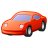Creator

Add a variable with this expression: This basically calculates (Total SF - Unallocated) and doesn't change with selections.Use the variable in the chart.

=vVar*Num#(subfield([SF Percentage],'%',1))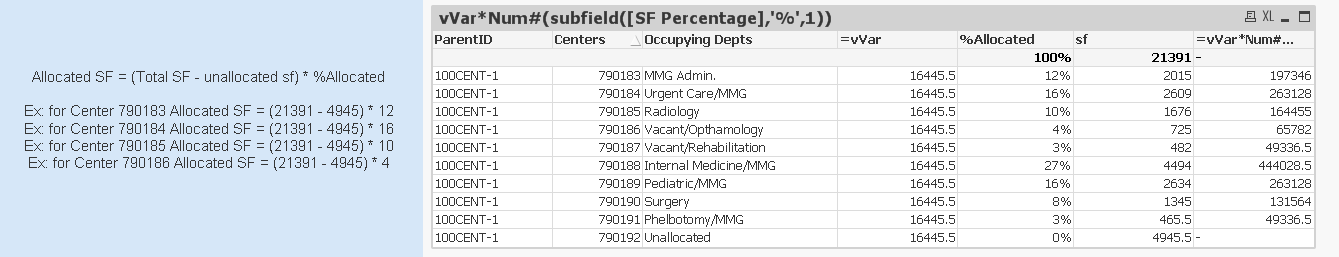Master III

Hi Ansh,

see Attachment.

Regards,

Antonio.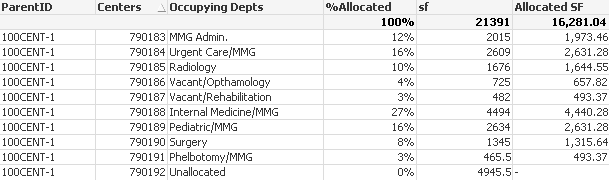Creator
Author

Thank you Antonio - But when I put this in Text box or do expression total it goes blank...can you please sujjest ?Master III

In Text Box

=Num(Sum(Aggr(
(Sum(TOTAL [Adj Net Size - SF])-Sum({<[Occupying Depts]={'Unallocated'}>} TOTAL [Adj Net Size - SF]))*
Num(Left([SF Percentage],5)),ParentID,Centers,[Occupying Depts])),'#,##0.00')